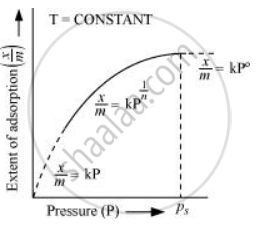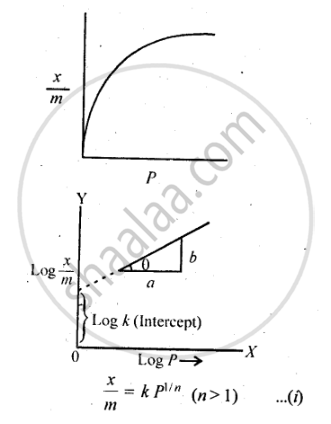Share

# Describe Freundlich Adsorption Isotherm. - CBSE (Science) Class 12 - Chemistry

#### Solution 1Freundlich adsorption isotherm gives an empirical relationship between the quantity of gas adsorbed by the unit mass of solid adsorbent and pressure at a specific temperature.

From the given plot it is clear that at pressure PS, x/m reaches the maximum valve. Ps is called the saturation pressure. Three cases arise from the graph now.

Case I- At low pressure:

The plot is straight and sloping, indicating that the pressure in directly proportional to x/m i.e., x/m alpha P

x/m = kP  (k is constant)

Case II- At high pressure:

When pressure exceeds the saturated pressure, x/m becomes independent of P values

x/m alpha P^@

x/m = kP^@

Case III- At intermediate pressure:

At intermediate pressure, x/m  depends on P raised to the powers between 0 and 1. This relationship is known as the Freundlich adsorption isotherm.

x/m alpha P^(1/n)

x/m = kP^(1/n)      n > 1

Now taking log: log  x/m =log k + 1/n log P

On plotting the graph between log (x/m) and log P, a straight line is obtained with the slope equal to 1/n and the intercept equal to log k#### Solution 2

Freundlich, in 1909, gave an empirical relationship between the quantity, of gas adsorbed by unit mass of solid adsorbent and pressure at a particular temperature. The relationship can be expressed by the following equation:where x is the mass of the gas adsorbed by mass ‘m’ of the adsorbent at pressure P, k and n are constants which depend on the nature of the adsorbent and the gas at a particular temperature. The relationship is generally represented in the form of a curve where mass of the gas adsorbed per gram by the adsorbent is plotted against pressure. These curves indicate that at a fixed pressure, there is a decrease in physical adsorption with increase in temperature. These curves always seem to approach saturation at high pressure.
Taking log of equation (i), we get

log  x/m =log k + 1/n log P

Is there an error in this question or solution?

#### APPEARS IN

NCERT Solution for Chemistry Textbook for Class 12 (2018 to Current)
Chapter 5: Surface Chemistry
Q: 5.2 | Page no. 146